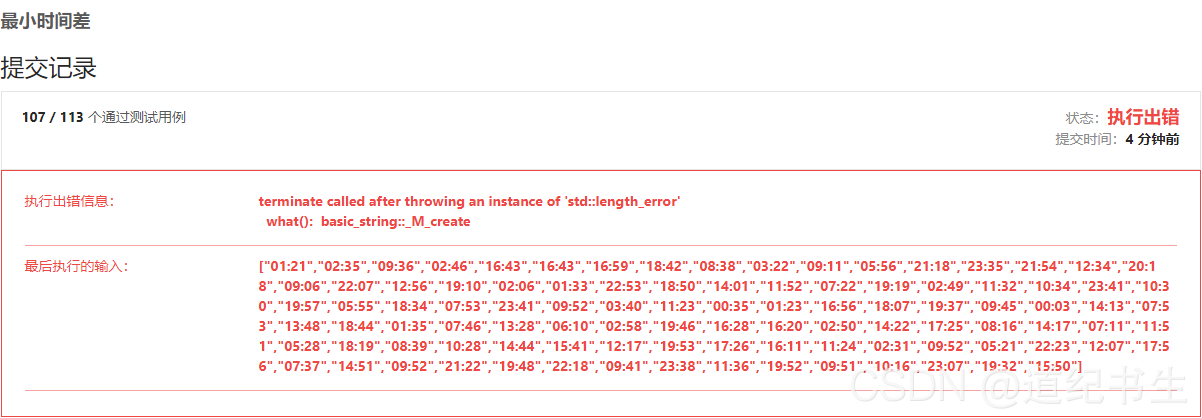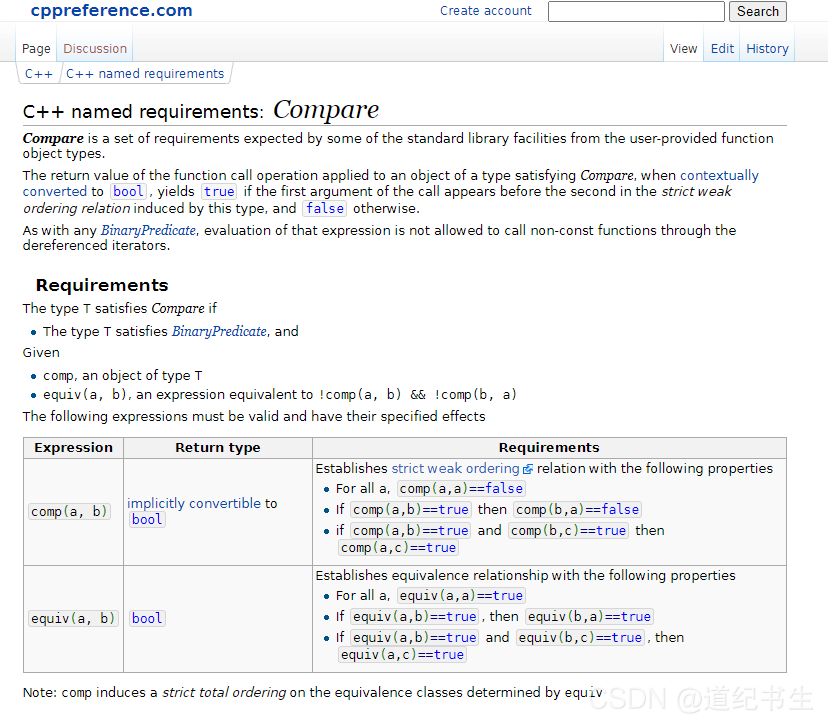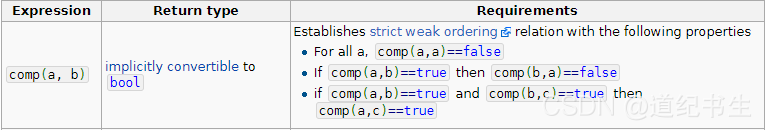# C++: terminate called after throwing an instance of ‘std::length_error‘ (sort function cmp sorting rules problem)

Leetcode-539: encountered a big problem when brushing the minimum time difference:Errors are reported as follows:

``````terminate called after throwing an instance of 'std::length_error'
what():  basic_string::_M_create``````

The error code is as follows:

``````class Solution {
public:
static bool cmp(string x,string y){ //Handwritten sorting rules Sort by chronological ascending order
int Xshi= (x-'0')*10+x-'0';
int Xfen= (x-'0')*10+x-'0';
int Yshi= (y-'0')*10+y-'0';
int Yfen= (y-'0')*10+y-'0';
if(Xshi>Yshi || (Xshi==Yshi && Xfen> Yfen))  return false;
else return true;
}
//input time has been sorted x is less than y to find two time minute difference function
int Time_diff(string x,string y){
int Xshi= (x-'0')*10+x-'0';
int Xfen= (x-'0')*10+x-'0';
int Yshi= (y-'0')*10+y-'0';
int Yfen= (y-'0')*10+y-'0';

int diff=(Yshi-Xshi)*60;
if(Yfen>Xfen) diff+=Yfen-Xfen;
else diff-=Xfen-Yfen;
return diff;
}
int findMinDifference(vector<string>& timePoints) {
//After sorting, compare the two adjacent to each other and also compare the first and last
sort(timePoints.begin(),timePoints.end(),cmp);
//compare the first and the last
int T_min=min(  Time_diff(timePoints,timePoints[timePoints.size()-1]),
1440-(Time_diff(timePoints,timePoints[timePoints.size()-1])) );
//Two adjacent to each other for comparison
for(int i=1;i<timePoints.size();i++)
{
T_min=min(T_min, Time_diff(timePoints[i-1],timePoints[i]) );
}
return T_min;
}
};
``````

Looking back and forth at the code, I was stunned that I couldn’t see any problems. I consulted hundreds of relevant web pages, basically for the following two reasons:

1. an empty string was entered. The array subscript is out of bounds

However, these two problems are obviously not in my code. Finally, I checked all night and found the official description of sort sorting comparison.

https://en.cppreference.com/w/cpp/named_req/CompareLet’s focus here:In other words, for the CMP comparison function we handwritten, the following three points should be met:

1. for all input a, input two identical elements, namely CMP (a, a). If the returned result is false, it cannot be false. If the result of entering CMP (a, b) is true, conversely, the result of entering CMP (B, a) is false, that is, if a > b. No more B > a. Otherwise, there will be contradictions. If the result of input CMP (a, b) is true, and the result of input CMP (B, c) is true, then the result of CMP (a, c) should also be true, which is also well understood. If a > B and B > C then a must be greater than C

Here, we should mainly pay attention to the first point. When the two numbers are the same, the returned result should be false, and my code:

``````    static bool cmp(string x,string y){ //Handwritten sorting rules Sort by chronological ascending order
int Xshi= (x-'0')*10+x-'0';
int Xfen= (x-'0')*10+x-'0';
int Yshi= (y-'0')*10+y-'0';
int Yfen= (y-'0')*10+y-'0';
if(Xshi>Yshi || (Xshi==Yshi && Xfen> Yfen))  return false;
else return true;
}``````

This rule is not satisfied. So add one   =  Number to make it comply with the rules.

``````    static bool cmp(string x,string y){ //Handwritten sorting rules Sort by chronological ascending order
int Xshi= (x-'0')*10+x-'0';
int Xfen= (x-'0')*10+x-'0';
int Yshi= (y-'0')*10+y-'0';
int Yfen= (y-'0')*10+y-'0';
if(Xshi>Yshi || (Xshi==Yshi && Xfen>= Yfen))  return false;
else return true;
}``````

The problem that bothered me all night was solved.